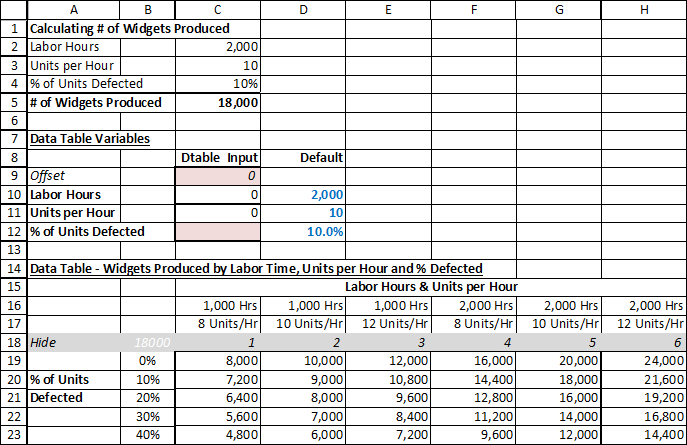# How to Create a Three Variable Data Table in Excel?

### Introduction

The key to making a three-variable data-table (or any higher number of variables, such as 4, 5, etc.) is to use the offset function to populate a set of values into the base calculation. (The data-table’s constraint of only having two variables remain unchanged.)### Setting Up the Model

There are three parts to this example:

1. Rows 1 to 5. “Calculating # of Widgets Produced”:
• This is the “base model”, where the actual calculation occurs.
• # of Widgets Produced = labour hours times units per hour times % of units defected. Cells C2, C3 and C4 are possible inputs.
• C5 is the output we are looking for (# of Widgets Produced).
• Remember that all the data-table does is feed different possible input values to get answers for each scenario.
2. Rows 7 to 12. “Data-Table Variables”: This is where the data table change actual cells to create different scenarios.
3. Rows 14 to 23. “Data Table – Widgets Produced by Labor Time, Units per Hour, and % Defected”

### Step by Step Explanation

1. Data table changes cells C9, C12
2. Row 18 are possible values for C9. (1, 2, 3, 4..6) Notice that these are not the actual values to be used to calculate # of widgets produced. Instead, C10 and C11 are actual values to calculate # of widgets produced. C10 and C11 uses C9’s value as the offset to pick up the correct hours and # units/hour values on rows 16 and 17.
• [C10] = OFFSET(\$B\$16,0,\$C\$9)
Starting from Cell B16, Excel is to go to the cell on the right based on the value of Cell C9. So if C9 is 0, stay on current cell. If C9 is 3 then go 3 to the right, meaning E9.
• [C11] = OFFSET(\$B\$17,0,\$C\$9)
Similar logic.
3. B19 to B23 are possible values for C12. (0%, 10%..40% Actual % values of defects.)
4. D10, D11, D12 are defaults. This is what the formula in rows 2 to 5 show on the screen by default.
• [C2] =IF(‘3-Var Datatable’!C9=0,D10,’3-Var Datatable’!C10)
This means if C9=0 (by default it should be 0, since only the data table changes C9), formula should equal to the default “Labour Hours” value (stored in D10). Otherwise, if value is no 0 (meaning data table is calculating value), then it will use cell C10, which is some input value based on the data table.
• [C3], [C4] is similar logic.

### How to Change this Model for Your Purposes

1. To use this model first you need to decide on the “Base model”. This includes:
1. The required formula inputs
2. The output you are looking to calculate
3. And the formula of calculation.
2. Input the default values for input cells – this is the base case.
3. Decide on the combination of inputs that you want to perform the sensitivities on.

1. There is no real limitations on the combinations, except consideration for how much data you want to present to the reader. You may want to be very comprehensive or brief depending on what you are using the file for.
2. In the example above, we applied # of hours and units per hour as the column input. You can, of course, change that to fit your inputs.
4. Decide on the values of inputs you want to calculate in the data table. In this example:
1. The Labour Hours & Units per Hour section shows 1,000 and 2,000 hours combined with 8, 10, and 12 units per hour, resulting in 6 possible “scenarios”. It is possible to provide more granularity for labour hours (e.g. adding 1,500 hours), # of units per hour, etc.
2. The % of units defected has a high range here (0% to 40%), as this is only an example to show you how a 3-variable data table can work. For practical purposes, you may want to narrow the range and provide better detail. Once again, the range of data table input values depends what type of situation you are dealing with.
5. Make sure cell B18 is linked to cell C5 or the output cell for the “Base Model” section.